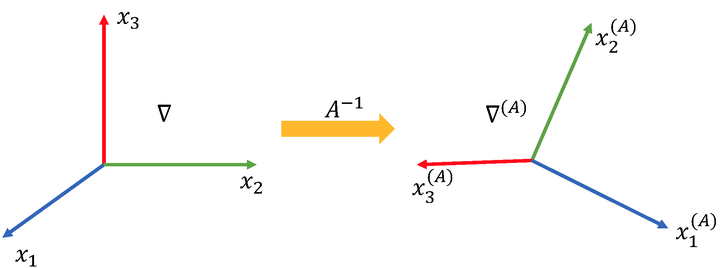# PDO-eConvs: Partial Differential Operator Based Equivariant Convolutions### Abstract

Recent research has shown that incorporating equivariance into neural network architectures is very helpful, and there have been some works investigating the equivariance of networks under group actions. However, as digital images and feature maps are on the discrete meshgrid, how to preserving strong equivariance in the discrete domain is still very challenging. In this work, we solve this problem in an elegant way. To be specific, we parameterize conventional convolutional filters as a linear combination of PDOs, and use these steerable filters to design a system equivariant to a very general continuous group, the n-dimension Euclidean group, E(n). In implementation, we discretize the system using the numerical schemes of PDOs. Theoretically, the approximation error of PDO-eConvs is of the quadratic order, and it is the first time that the error analysis is provided when the equivariance is approximate. Extensive experiments on rotated MNIST and natural image classification show that PDO-eConvs perform competitively yet use parameters much more efficiently.

Type
Publication
International Conference on Machine Learning